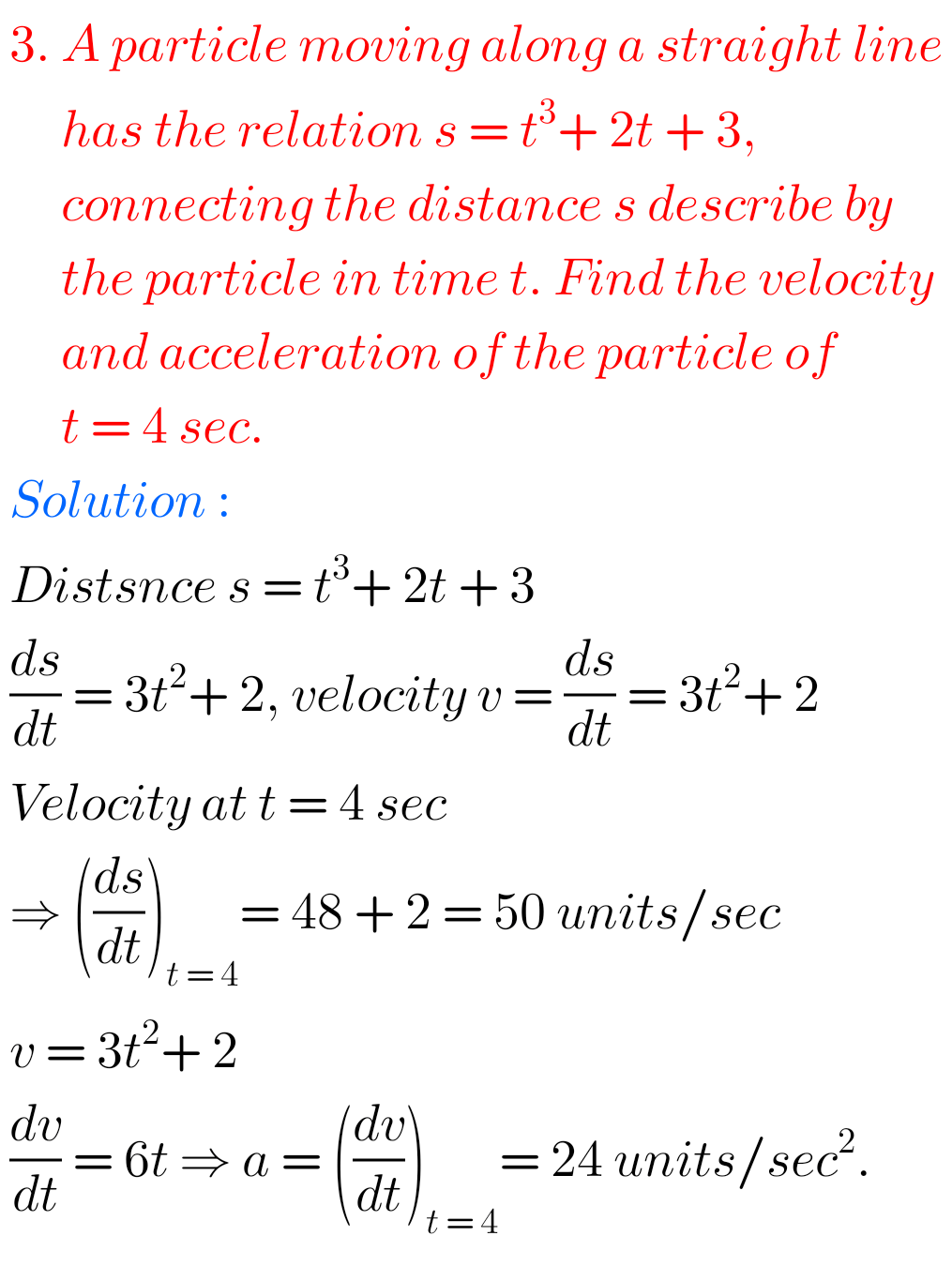## Solutions for Applications of Derivatives exercise 10(e)

Inter Maths 1Bsolutions Applications of Derivatives exercise 10(e) Intermediate Mathematics 1B Applications of Derivatives exercise 10(e) textbook solutions are given. Study the lesson Applications of Derivatives very well. Practice the example problems and solutions given in the textbook Observe the given below solutions and try them in your own method You can also see Inter …